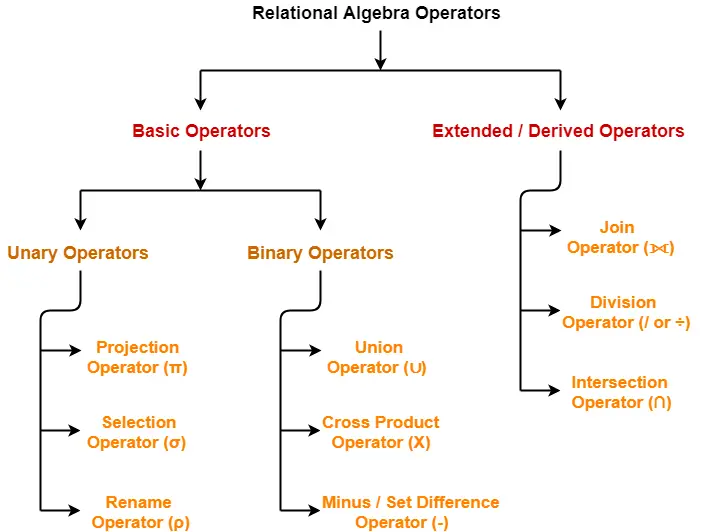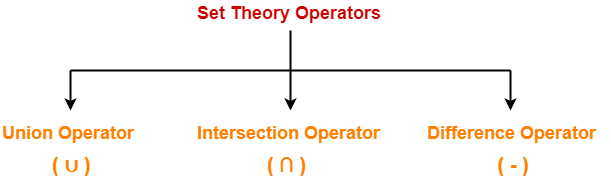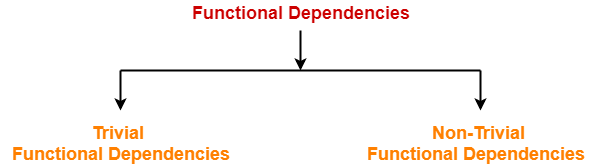## Relational Algebra Operators-

Before you go through this article, make sure that you have gone through the previous article on Introduction to Relational Algebra.

The operators in relational algebra are classified as-## Set Theory Operators-

Following operators are called as set theory operators-1. Union Operator (∪)
2. Intersection Operator (∩)
3. Difference Operator (-)

### Condition For Using Set Theory Operators

To use set theory operators on two relations,

The two relations must be union compatible.

Union compatible property means-

• Both the relations must have same number of attributes.
• The attribute domains (types of values accepted by attributes) of both the relations must be compatible.

Also read- Selection Operator and Projection Operator

## 1. Union Operator (∪)-

Let R and S be two relations.

Then-

• R ∪ S is the set of all tuples belonging to either R or S or both.
• In R ∪ S, duplicates are automatically removed.
• Union operation is both commutative and associative.

## Example-

Consider the following two relations R and S-

 ID Name Subject 100 Ankit English 200 Pooja Maths 300 Komal Science

#### Relation R

 ID Name Subject 100 Ankit English 400 Kajol French

#### Relation S

Then, R ∪ S is-

 ID Name Subject 100 Ankit English 200 Pooja Maths 300 Komal Science 400 Kajol French

## 2. Intersection Operator (∩)-

Let R and S be two relations.

Then-

• R ∩ S is the set of all tuples belonging to both R and S.
• In R ∩ S, duplicates are automatically removed.
• Intersection operation is both commutative and associative.

## Example-

Consider the following two relations R and S-

 ID Name Subject 100 Ankit English 200 Pooja Maths 300 Komal Science

#### Relation R

 ID Name Subject 100 Ankit English 400 Kajol French

#### Relation S

Then, R ∩ S is-

 ID Name Subject 100 Ankit English

## 3. Difference Operator (-)-

Let R and S be two relations.

Then-

• R – S is the set of all tuples belonging to R and not to S.
• In R – S, duplicates are automatically removed.
• Difference operation is associative but not commutative.

### Example-

Consider the following two relations R and S-

 ID Name Subject 100 Ankit English 200 Pooja Maths 300 Komal Science

#### Relation R

 ID Name Subject 100 Ankit English 400 Kajol French

#### Relation S

Then, R – S is-

 ID Name Subject 200 Pooja Maths 300 Komal Science

#### Relation R – S

Get more notes and other study material of Database Management System (DBMS).

Watch video lectures by visiting our YouTube channel LearnVidFun.

## Relational Algebra Operators-

Before you go through this article, make sure that you have gone through the previous article on Introduction to Relational Algebra.

The operators in relational algebra are classified as-## Projection Operator-

• Projection Operator (π) is a unary operator in relational algebra that performs a projection operation.
• It displays the columns of a relation or table based on the specified attributes.

 π(R)

## Example-

Consider the following Student relation-

 ID Name Subject Age 100 Ashish Maths 19 200 Rahul Science 20 300 Naina Physics 20 400 Sameer Chemistry 21

Then, we have-

### Result for Query πName, Age(Student)-

 Name Age Ashish 19 Rahul 20 Naina 20 Sameer 21

### Result for Query πID , Name(Student)-

 ID Name 100 Ashish 200 Rahul 300 Naina 400 Sameer

## Point-01:

• The degree of output relation (number of columns present) is equal to the number of attributes mentioned in the attribute list.

## Point-02:

• Projection operator automatically removes all the duplicates while projecting the output relation.
• So, cardinality of the original relation and output relation may or may not be same.
• If there are no duplicates in the original relation, then the cardinality will remain same otherwise it will surely reduce.

## Point-03:

• If attribute list is a super key on relation R, then we will always get the same number of tuples in the output relation.
• This is because then there will be no duplicates to filter.

## Point-04:

• Projection operator does not obey commutative property i.e.

π <list2> (π <list1> (R)) ≠ π <list1> (π <list2> (R))

## Point-05:

• Following expressions are equivalent because both finally projects columns of list-1

π <list1> (π <list2> (R)) = π <list1> (R)

## Point-06:

• Selection Operator performs horizontal partitioning of the relation.
• Projection operator performs vertical partitioning of the relation.

## Point-07:

• There is only one difference between projection operator of relational algebra and SELECT operation of SQL.
• Projection operator does not allow duplicates while SELECT operation allows duplicates.
• To avoid duplicates in SQL, we use “distinct” keyword and write SELECT distinct.
• Thus, projection operator of relational algebra is equivalent to SELECT operation of SQL.

Next Article- Set Theory Operators in Relational Algebra

Get more notes and other study material of Database Management System (DBMS).

Watch video lectures by visiting our YouTube channel LearnVidFun.

## Relational Algebra Operators-

Before you go through this article, make sure that you have gone through the previous article on Introduction to Relational Algebra.

The operators in relational algebra are classified as-## Selection Operator-

• Selection Operator (σ) is a unary operator in relational algebra that performs a selection operation.
• It selects those rows or tuples from the relation that satisfies the selection condition.

 σ(R)

## Examples-

• Select tuples from a relation “Books” where subject is “database”

σsubject = “database” (Books)

• Select tuples from a relation “Books” where subject is “database” and price is “450”

σsubject = “database” ∧ price = “450” (Books)

• Select tuples from a relation “Books” where subject is “database” and price is “450” or have a publication year after 2010

σsubject = “database” ∧ price = “450” ∨ year >”2010″ (Books)

## Point-01:

• We may use logical operators like ∧ , ∨ , ! and relational operators like  = , ≠ , > , < , <= , >= with the selection condition.

## Point-02:

• Selection operator only selects the required tuples according to the selection condition.
• It does not display the selected tuples.
• To display the selected tuples, projection operator is used.

## Point-03:

• Selection operator always selects the entire tuple. It can not select a section or part of a tuple.

## Point-04:

• Selection operator is commutative in nature i.e.

σ A ∧ B (R) = σ B ∧ A (R)

OR

σ (σ A(R)) = σ (σ B(R))

## Point-05:

• Degree of the relation from a selection operation is same as degree of the input relation.

## Point-06:

• The number of rows returned by a selection operation is obviously less than or equal to the number of rows in the original table.

Thus,

• Minimum Cardinality = 0
• Maximum Cardinality = |R|

Next Article- Projection Operation in Relational Algebra

Get more notes and other study material of Database Management System (DBMS).

Watch video lectures by visiting our YouTube channel LearnVidFun.

## Relational Algebra-

 Relational Algebra is a procedural query language which takes a relation as an input and generates a relation as an output.

## Relational Algebra Operators-

The operators in relational algebra may be classified as-We will discuss all these operators one by one in detail.

## Characteristics-

Following are the important characteristics of relational operators-

• Relational Operators always work on one or more relational tables.
• Relational Operators always produce another relational table.
• The table produced by a relational operator has all the properties of a relational model.

Next Article- Selection Operator in Relational Algebra

Get more notes and other study material of Database Management System (DBMS).

Watch video lectures by visiting our YouTube channel LearnVidFun.

## Functional Dependency-

 In any relation, a functional dependency α → β holds if- Two tuples having same value of attribute α also have same value for attribute β.

Mathematically,

If α and β are the two sets of attributes in a relational table R where-

• α ⊆ R
• β ⊆ R

Then, for a functional dependency to exist from α to β,

If t1[α] = t2[α], then t1[β] = t2[β]

 α β t1[α] t1[β] t2[α] t2[β] ……. …….

 fd : α → β

## Types Of Functional Dependencies-

There are two types of functional dependencies-1. Trivial Functional Dependencies
2. Non-trivial Functional Dependencies

## 1. Trivial Functional Dependencies-

• A functional dependency X → Y is said to be trivial if and only if Y ⊆ X.
• Thus, if RHS of a functional dependency is a subset of LHS, then it is called as a trivial functional dependency.

### Examples-

The examples of trivial functional dependencies are-

• AB → A
• AB → B
• AB → AB

## 2. Non-Trivial Functional Dependencies-

• A functional dependency X → Y is said to be non-trivial if and only if Y ⊄ X.
• Thus, if there exists at least one attribute in the RHS of a functional dependency that is not a part of LHS, then it is called as a non-trivial functional dependency.

### Examples-

The examples of non-trivial functional dependencies are-

• AB → BC
• AB → CD

## Inference Rules-

### Reflexivity-

If B is a subset of A, then A → B always holds.

### Transitivity-

If A → B and B → C, then A → C always holds.

### Augmentation-

If A → B, then AC → BC always holds.

### Decomposition-

If A → BC, then A → B and A → C always holds.

### Composition-

If A → B and C → D, then AC → BD always holds.

If A → B and A → C, then A → BC always holds.

## Rules for Functional Dependency-

### Rule-01:

A functional dependency X → Y will always hold if all the values of X are unique (different) irrespective of the values of Y.

### Example-

Consider the following table-

 A B C D E 5 4 3 2 2 8 5 3 2 1 1 9 3 3 5 4 7 3 3 8

The following functional dependencies will always hold since all the values of attribute ‘A’ are unique-

• A → B
• A → BC
• A → CD
• A → BCD
• A → DE
• A → BCDE

In general, we can say following functional dependency will always hold-

 A → Any combination of attributes A, B, C, D, E

Similar will be the case for attributes B and E.

### Rule-02:

A functional dependency X → Y will always hold if all the values of Y are same irrespective of the values of X.

### Example-

Consider the following table-

 A B C D E 5 4 3 2 2 8 5 3 2 1 1 9 3 3 5 4 7 3 3 8

The following functional dependencies will always hold since all the values of attribute ‘C’ are same-

• A → C
• AB → C
• ABDE → C
• DE → C
• AE → C

In general, we can say following functional dependency will always hold true-

 Any combination of attributes A, B, C, D, E → C

Combining Rule-01 and Rule-02 we can say-

 In general, a functional dependency α → β always holds- If either all values of α are unique or if all values of β are same or both.

### Rule-03:

For a functional dependency X → Y to hold, if two tuples in the table agree on the value of attribute X, then they must also agree on the value of attribute Y.

### Rule-04:

For a functional dependency X → Y, violation will occur only when for two or more same values of X, the corresponding Y values are different.

Next Article- Equivalence of Functional Dependencies

Get more notes and other study material of Database Management System (DBMS).

Watch video lectures by visiting our YouTube channel LearnVidFun.